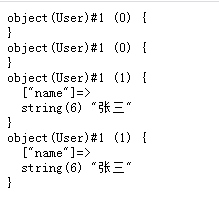• 主要介绍了Python引用传值概念与用法,简单分析了Python引用传值的概念、功能并结合实例形式总结分析了Python引用传值的具体实现与使用方法,需要的朋友可以参考下
• java引用传值 package com.mpw; /** * 引用传值 两数交换 * @author anneli * @date 2019年9月23日 下午3:39:00 * */ public class Swap { public static void swap(MyClass a, MyClass b) { int tmp =...
 java引用传值

package com.mpw;

/**
* 引用传值 两数交换
* @author anneli
* @date 2019年9月23日 下午3:39:00
*
*/
public class Swap {

public static void swap(MyClass a, MyClass b) {
int tmp = a.getNum();
a.setNum(b.getNum());
b.setNum(tmp);
}

public static void main(String[] args) {
MyClass a = new MyClass(11);
MyClass b = new MyClass(22);
Swap.swap(a, b);
System.out.println(a.getNum() + " " + b.getNum());
}
}

class MyClass {
int Num;

public MyClass(int Num){
this.Num = Num;
}

public int getNum() {
return Num;
}

public void setNum(int tmp) {
Num = tmp;
}
}


展开全文java
• PHP引用传值实例PHP引用传值实例 PHP引用传值实例 下面展示一些 内联代码片。 // A code block var foo = 'bar'; /* * 获取完整的地区名称 */ public function getRegionName(RegionRepository $... PHP引用传值实例PHP引用传值实例 PHP引用传值实例 下面展示一些 内联代码片。 // A code block var foo = 'bar';   /* * 获取完整的地区名称 */ public function getRegionName(RegionRepository$regionRepository)
{
try {
$rule = [ 'cri_code' => 'require', ];$msg = [
'cri_code.require' => 'cri_code can not be empty',
];
$validate = Validate::make($rule, $msg);$result = $validate->check($this->request->post());
if (!$result) { return api_format(self::PARAM_ERROR,$validate->getError());
}

$criCode =$this->request->post('cri_code');
$info =$regionRepository->getRegionByCriCode($criCode); if (!$info) {
return api_format(self::SYSTEM_ERROR, 'System error');
}
$prefix = '';$regionRepository->parentRegionName($info->cri_parent_code,$prefix);

$data['cri_name'] =$prefix . $info->cri_name; return api_format(self::OK, 'Success',$data);
} catch (Exception $e) { throw$e;
}
}

下面引用传值的方法。
参数$string既可以说是入参也可以说是出参，即它的值是最新的值。   /* * 获取完整地区名称(递归方法之：引用传值) */ public function parentRegionName($criCode, &$string) { try { if ($criCode != '000000') {
$parentRegion = self::getRegionByCriCode($criCode);
if (!$parentRegion) { return$string;
}
$string =$parentRegion->cri_name . $string; self::parentRegionName($parentRegion->cri_parent_code, $string); } /*-----------------以下是非引用传值的写法---------------------*/ // if ($criCode != '000000') {
//                $parentRegion = self::getRegionByCriCode($criCode);
//                if (!$parentRegion) { // return$string;
//                }
//                $string =$parentRegion->cri_name . $string; // return self::parentRegionName($parentRegion->cri_parent_code, $string); // } // // return$string;
} catch (Exception $e) { throw$e;
}
}



展开全文php linux
• 构造函数,借助构造函数 解释 【赋值传值】，【引用传值】，【指针传值】
• 对象传值本质上是引用传值，将一个对象变量（a）赋值给另个变量（a）赋值给另个变量（a）赋值给另个变量（b），实际上是将a存储的对象内存引用地址赋值a存储的对象内存引用地址赋值a存储的对象内存引用地址赋值b，...

对象传值本质上是引用传值，将一个对象变量（$a）赋值给另个变量（$b），实际上是将$a存储的对象内存引用地址赋值$b，此时两个变量指向的就是一个对象。其中一个变量发送改变，另一个也会跟着改变。和引用变量一个道理。

1. 对象传值示例

对象传值本质上就是引用传值
$a = new User;$b = $a;//对象传值 var_dump($a, $b);$b->name = '张三';

var_dump($a,$b);

class User
{

}

运行结果，其实第一次打印就可以看出来$a 和 $b 是一个对象，因为对象标识符一样（都是 1）2. 解释说明

如果将一个对象赋值给变量（$a），$a实际上存的是对象的内存引用地址，而不是对象
对象存在堆内存中，内存引用地址存在栈内存中，所以将 $a 赋值给另一个变量 $b，
实际上是将 $a 存的对象的内存引用地址赋值给了$b，也就是 $a 和$b 存的是同一个引用地址，
所以两个变量实际上是一个对象，因此 $b 发生改变，$a 也跟着改变


展开全文•代码
• foreach($arr as &$v){ // 引用传值, 结束后, $arr和$v之间的引用关系并没有断开 // 再次调用时要注意, 或者直接unset($v) } foreach($arr as &$v){ // 引用传值, 结束后,$arr和$v之间的引用关系并没有断开 // 再次调用时要注意, 或者直接unset($v)
}
展开全文• 引用传值与指针传值其实没有区别，本质都是传址int* ADD(int A,int B) { int C = A + B; return &c; } int main() { int a = 1,b = 2; int *c = ADD(a,b); int d = 5; printf("%d",d); printf("%d",*c); }...
• 引用传值，允许被调用的函数能够访问调用函数内的变量，即在被调函数内修改参数值，主调函数内的值也跟着发生变化，因为二者是同一块地址。 如上图所示，在调用者函数——main函数中声明了一个times变量，并赋值...
• 碰到了一个群内求助关于引用传值的问题. 这里把容易混淆的赋值和类传值问题进行详细说明 引用修改 @Test public void testEquals() { Boolean b = new Boolean(false); change(b); System.out.println(b...
• foreach 引用传值& php 怎么在foreach中循环数组 ，的时候添加元素跟值 foreach($arr as$key => &$vo){ //注意，由于上面遍历的时候写了地址传值符&, //所以下面可以直接给$vo 赋值;如果不...
• 二话不说，先上代码： function callBySharing(k){ k.push(1); } m = []; callBySharing(m);...因为在调用中， m对于函数callBySharing（） 来说是可见的，所以最后会输出[1...还有一个就是 引用传值，int change(a, *bjavascript c
• PHP的引用传值 function change_value($num){$num+=2; } $age = 3; change_value(&$age);...CS的引用传值 static void Main(string[] args) { int age = 3; Console.WriteLin...
• php赋值有两种方式 1.传值赋值 2.引用赋值 &lt;?php //php传值方式 //传值赋值(两个人看两台电视) $a = 'zhangsan';... echo$a, '-', $b .... //引用传值(两个人看一台电视)$a = 'lisi';...PHP基础知识
• 学习了Python后，感觉到对Python里的引用传值有些疑惑，搜集了一些资料，同时编了一些实例，在此对引用传值解惑一下，分享给大家。 在Python里，一切事物皆为对象，并且规定参数的传递都是对象的引用。python参数...python 传参 值传递
• Java除了基本类型，其它都是引用传值，传值是改变变量引用的方向
• 1）当变量是一个对象（例如：{}或[]），使用 a = b = {} 这种形式传值的话，会变成会用传值，修改 a 时 b 会相应变化，修改 b 时 a 也一样会相应变化 ...2）当变量为数值型的话，则不会引用传值 var a = b = 0; ...
• 关于对象的引用传值 <?php $a = new stdClass();$b = $a;$a->age = 18; $b->age = 20; echo$a->age; 此时输出什么？如何使$a和$b相互不影响。(答案：20，$b = clone$a; )PHP面试
• Python函数的参数传值使用的是引用传值，也就是说传的是参数的内存地址值，因此在函数中改变参数的值，函数外也会改变。 这里需要注意的是如果传的参数类型是不可改变的，如String类型、元组类型，函数内如需改变...
• 引用传值,传引用后,是不同的地址名称,但都指向同一个内存实体;改变其中一个,另外一个就也被改变; 以下我将通过三个列子来详细讲解这两个传值的区别: Example1： <?php //普通传值 $param1=1; ... • python 数组 A=B 默认的是引用传值，改变A中的元素，B中的元素同样改变。 而A=B[:]则是传值，改变A中的元素的值，B中元素的值保持不变。 样例： 引用 a=[1,2,3,4] b=a[:] b=4 print(b) print(a) def Cal(A)... • import java.util.ArrayList; import java.util.List;... * String不是引用传值，List等对象是引用传值。 因为String不是引用传值，因此changeStr(String old)中的操作不影响外界。 * */ public class T... • 在php中使用引用赋值只需要在原始对象前加个&就可以了，具体休怎么用呢，可以看下文解释和实例 • 在变量赋值时使用引用传值，那么，这两个变量就会使用同一个存储地址，相互影响。示例如下： <?php$a = 12; $b = &$a; echo $a; // 12 echo$b; // 12 $b = 13; echo$a; // 13 echo $b; // 13 将变量 a ...php 后端 • 关于引用传值和值传递的理解 概念： 值传递：变量值在函数内的任何改变在函数外都被忽略 引用传值：变量值在函数内的任何改变在函数外生效 下面函数$name为值传递，&$sex为引用传值 function func($name......